# All About Time-Weighted Average Price (TWAP)

TWAP or Time-Weighted Average Price is commonly used as an order execution strategy to perform huge trades. It is done by breaking the trades into equal parts to minimize signaling and slippage.

##### What is Time-Weighted Average Price (TWAP)

TWAP is a calculation that determines the weighted average price over a specified period. Traders use TWAP to place large orders without excessively impacting the market price. This trading strategy is executed by breaking down large orders into smaller orders priced near TWAP.

So, if a trader wants to purchase 20,000 shares, the trader could choose to buy 1,000 shares every 20 minutes for 6 hours and 20 minutes.

Here’s the TWAP chart: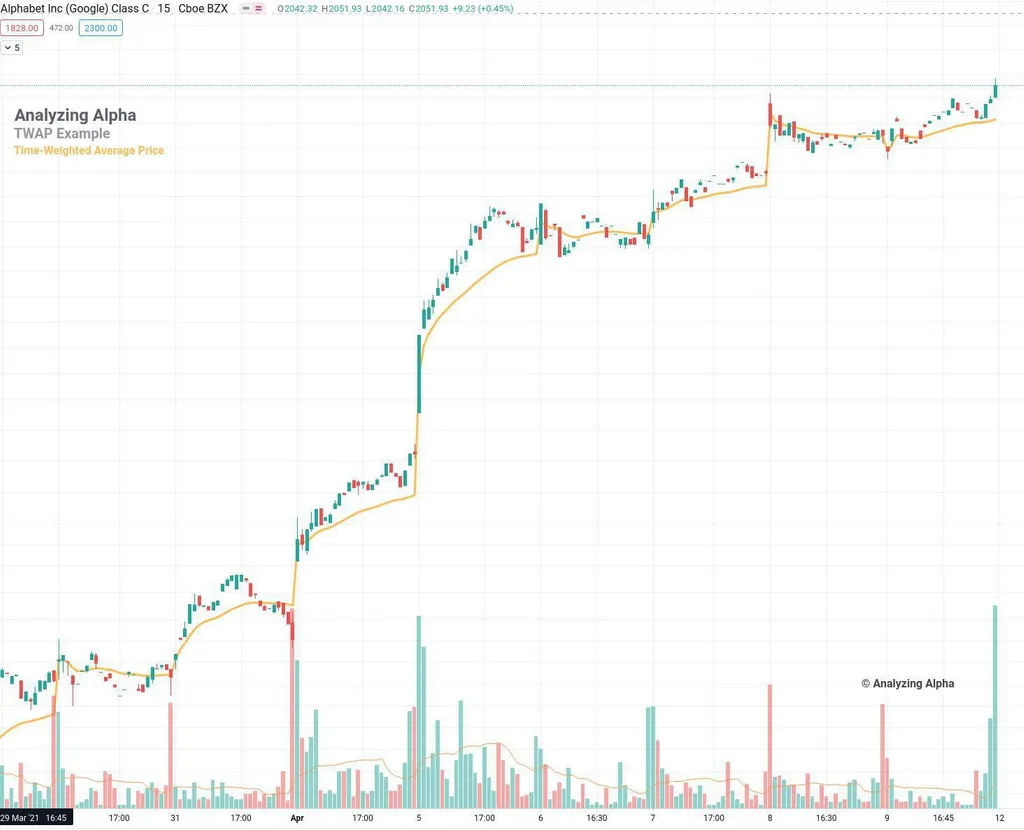TWAP is similar to VWAP (Volume-Weighted Average Price), but in TWAP, there is no volume element in its calculation.

##### Reasons to Use TWAP

Here are a few reasons why traders use TWAP:

• By breaking down large orders, traders can minimize the impact of a large order on the market price.
• TWAP is a good alternative to VWAP because volume isn’t linear. TWAP is best used on higher-volume securities.
##### TWAP Formula

To calculate TWAP, you need to take the average “typical price” for n periods. Use the open, high, low, and close when factoring in the typical price.

tp = (Low +Close + High) / 3

TWAP = (TP_1 + TP_2 + TP_n) / n

The calculation is not complex at all.

When you get the TWAP, the order price is compared to determine if the security is overvalued or undervalued. It is undervalued if the order price is below the TWAP and overvalued if it is more than the TWAP.

##### Calculating TWAP in Python

Here’s how to do the calculation of TWAP in Python: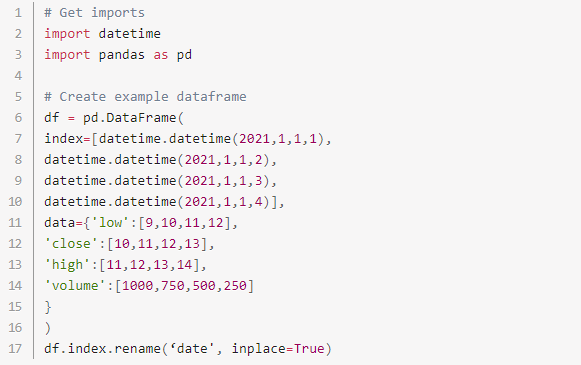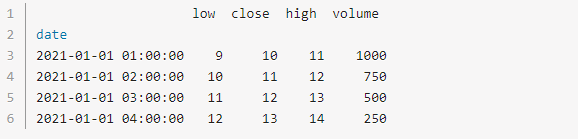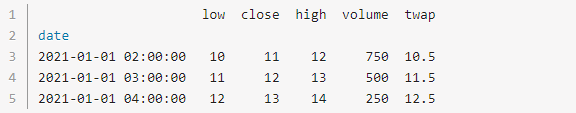Here are some of the benefits of using TWAP:

• Simplicity – The execution algorithm is very simple.
• Risk Mitigation – It reduces both slippage and signaling risk.

One primary drawback is signaling risk. Trading in a predictable pattern may signal risk for orders that take up a noticeable daily volume percentage.

Suboptimal Intraday Execution is another drawback. See this SPY chart below, where you will see the peaks at the open and the close.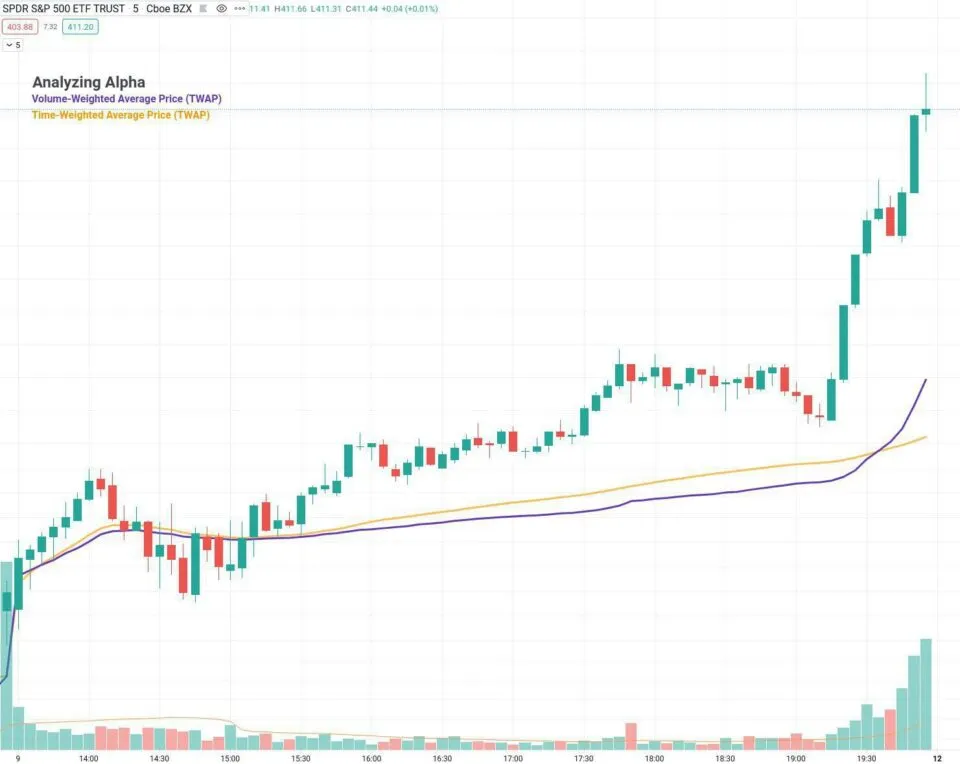##### TWAP vs. VMWA vs VWAP

Let’s take a look at how TWAP differs from VMWA and VWAP.

##### Definition
• TWAP – Time-Weighted Average Price; a calculation that determines the weighted average price of an asset over a period.
• VWMA – Volume Weight Moving Average; a moving average; that assigns a different weight to each closing price.
• VWAP – Volume Weighted Average Price; a calculation that computes the average based on the number of shares traded at different prices throughout the trading day divided by the total number of shares transacted.
##### Component
• TWAP – It focuses on the price and time.
• VWMA – It calculates the average closing prices with respect to the volume.
• VWAP – It is based on time and volume.
##### Objective
• TWAP – It spreads smaller trades over a specific period, lowering its impact on the broader market.
• VWMA – It aims to determine emerging trends and confirm existing trends by studying the price and volume pattern.
• VWAP – It is used to determine an attractive price and profitable entry and exit points.
##### Formula
• TWAP – TWAP= ((TP1+TP2+….TPn)/n), where tp= (Low +Close + High) / 3
• VWMA – (C1V1 + C2V2 + C3*V3) / (V1+ V2+ V3), where C= closing price; V= volume
• VWAP – VWAP = (Typical Price x Volume) / cumulative volume.
##### Conclusion

TWAP (Time-Weighted Average Price) is a simple trading strategy used widely by traders because it prevents slippage and does not signal to other traders what move you’re making in the markets. You can easily learn and execute this strategy without any sweat!

want to learn how to algo trade so you can remove all emotions from trading and automate it 100%? click below to join the free discord and then join the bootcamp to get started today.